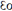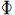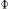×
Get Full Access to TTU - FIN 2401 - Study Guide - Midterm
Get Full Access to TTU - FIN 2401 - Study Guide - Midterm

×

TTU / Finance / FIN 2401 / What are the three properties of electric charge?

# What are the three properties of electric charge? Description

##### Description: This study guide covers the main concepts of Ch 23-26. I also added some formula shortcuts from the examples in the book. Hope this helps you! Good luck on the test!
11 Pages 7 Views 7 Unlocks
Reviews

Physics 2401-001 Test 1

## What are the three properties of electric charge?Material in the test: The test will cover Ch23-Ch26. The Test will have approximately 19-20  problems.

Material to bring to the test: Pencil(no pens because you will be using a scantron), 3x5 Notecard,  scientific calculator (make sure it works and possibly bring an extra set of batteries if you’re  prone to having bad luck), and an orange scantron

Note: For those of you who are taking this course with me, along with this study guide there is a  past exam on blackboard for study material. I would also suggest using the homework as study  material.

Note: For the test, know the material conceptually as well as mathematically. As per the exam on  Blackboard, some of the questions refer to the material as “ What would happen if…” and wants  you to think about the reaction and not just plug in numbers into the formulas on your note card.

## What does Coulomb's law state?Ch23

Ch 23.1 Properties of Electric Charges

Like charges repel; opposite charges attract

Electric charge is always conserved in an isolated system. Meaning , charge is transferred from  one object to another(NEVER created or destroyed).

When the two objects touch (known as charging by conduction) the total charge between the two  is divided evenly between the two

Protons(+) and electrons(-) have the same magnitude of charge but with different signs Neutrons have no charge

Ch 23.2 Charging Objects by Induction

Types of materials

-conductors: When charged, some of the charge distributes evenly throughout the material. Also  the general charge of the object, some of the charge moves freely. Ex. copper, aluminum, and  silver.

-insulators: when charged, the charge stays in the place of contact. Also the general charge of an  object, the charge stays in place. Ex. glass, rubber, and dry wood.

## What is electric field lines and its properties?-semiconductors: in-between the two extremes above. Ex. silicon, germanium

Induction

Charging by induction, or charging by not touching, is when you bring two objects together(one  usually charged positive or negative and without touching) and the charged object polarizes the  other. Don't forget about the age old question of dr goux

Polarizing an object means that the electrons and protons within an object are rearranged in a  way that protons are on one side and electrons on the other. Note: Think about how like charges  and unlike charges react to each other, this will tell you which sides the protons and electrons are  on Don't forget about the age old question of carl woese distinguished between the members of the archaea and the bacteria using studies of their

Ch 23.3 Coulomb’s Law

Formula

Fe = ke (|q1| |q2|)/r2 ???? This is for point charges

= ke (|q1| |q2|)/r2r^ ???? Vector form

Where

ke = 1/(4*pi*) = 9.0x109 Nm2/C2 ???? Coulomb’s Constant

εo = 8.854x10-12 C2/Nm2 Don't forget about the age old question of place the structures blood will pass through when precapillary sphincters are relaxed.

e = 1.602*10-19 aka “charge of the electron”

Particle(abrev.) Charge(Coulombs( C)) Mass(kg)

Electron(e) -1.602*10-19 (-e) 9.1094*10-31

Proton(p) 1.602*10-19 (e) 1.67262*10-27 Neutron(n) 0 1.67493*10-27

F1 = F21 + F31 + F41 ???? This describes the force on point 1 as it pertains to point 2,3, and 4 Remember when dealing with diagonal forces, break the force into its x and y components.If you want to learn more check out biol 191 unr

Ch 23.4 Analysis Model: Particle in a Field(Electric) E = F/q

where q is the charge

Fe = q*E

= ke(q1*q2)/r2 r^

So

E = ke(Q/r2) r^ ???? Vector Form, for a point charge

E = ke(2*x*Q) ???? for a electric dipole. Where ‘r’ was the hypotenuse and you   (y2+x2)3/2 replaced it with sqrt(y2+x2)

Ch 23.5 Electric Field of a Continuous Charge Distribution E = ke ∫ (dq/r2) r^ ???? Electric field due to a continuous charge distribution ρ = Q/V ???? Volume charge density. Units : C/m3

σ = Q/A ???? surface charge density. Units C/m3

λ = Q/ l ???? linear charge density. Units C/m

ρ = α *r ???? Spherically Symmetric where α is constant E = (ke*Q)/(a(l + a)) ???? Electric Field (EF) due to charged rod If you want to learn more check out ece 2040

Where ‘a’ is the distance the rod is from a point, ‘l ‘ is the

length of the rod

E = (ke*x*Q)/(a2+x2)3/2 ???? EF of a Uniform Ring of Charge

Where ‘a’ is the radius of the ring, ‘x’ is the length from the

ring to a point

E = 2*pi*ke*σ[1 – (x/(R2 + x2)1/2)]???? EF of a Uniformly Charged Disk

Ch 23.6 Electric Field Lines

Electric field lines(EFLs) point away from positive charges and towards negative charges. The more EFLs the higher the magnitude of E If you want to learn more check out 31 usc 3113

The number of EFLs is directly equivalent to the magnitude of the charge

EFLs never cross

Ch 23.7 Motion of a Charged Particle in a Uniform Electric Field Enet = σ/???? EF between two plates

F = q*E

F = m*a

So

a = (q*E)/m ???? acceleration of a particle

vf = sqrt((2qEd)/m) ???? final velocity for a positive particle between two charged plates  (one positive and one negative with the particle closer to the

positive plate)

Ch24

Ch 24.1 Electric Flux= E*A*cosθ = E*A ???? Net flux formula. Where θ is the angle between the EFL and the  normal(perpendicular to the surface) line. 2nd equal sign is when the

angle is equal to 90, making the EFLs point in the same direction as the

normal line.= ∫surface E*cosθ* dA ???? Net flux - closed surface, Units: N*m2/C

Ch 24.2 Gauss’s Law= Qin/???? Net flux through any closed surface surrounding point charge Q When the number of FLs going into a surface is equal to the FLs going out the charge is zero So

E*A = Qin/???? because both equations are equal toCh24.3 Application of Gauss’s Law to Various Charge Distributions Spherically Symmetric Charge Distribution

E = ke*Q/r2 ???? when r > a, ‘ r ‘ is the radius of the Gaussian sphere and ‘a’ is  the actual sphere

E = ke*Q*r/a3 ???? when r < a

Cylindrically Symmetric Charge Distribution

Einside = 2*ke* λ/r ???? when r > Ro, where ‘r’ is the Gaussian radius and ‘Ro’ is the radius of  an infinite length filament

Einside = ρ*r/(2*) ???? when r < Ro

A Plane of Charge

Eoutside = σ/(2*)

Einside = ρ*x/???? where ‘x’ is ½ the length of the Gaussian surface Esurface = ρ*d/(2*) ???? where ‘d’ is the width of the plane

Ch24.4 Conductors in Electrostatic Equilibrium

Properties of a Conductor in Electrostatic Equilibrium

∙ Inside a conductor, E = 0

∙ If the conductor is insolated and carries a charge, the charge resides on its surface ∙ E immediately outside a conductor is equal to σ/∙ For an irregularly shaped conductor, σ is greatest at locations where the radius of  curvature of the surface is smallest

∙ For equilibrium, Eout has to be perpendicular to the surface

A charged sphere inside a spherical conductor shell

E = ke*Q*r/a3 ???? inside the sphere, where ‘r’ = radius of Gaussian suface and  ‘a’ = radius of sphere

E = ke*Q/r2 ???? outside the sphere but inside the conductor shell E = 0 ???? inside the conductor shell

E = - ke*Q/r2 ???? outside the conductor shell

Ch25

Ch25.1 Electrical Potential and Potential Difference Wnet = ΔK ???? Work Energy Theorem

Fg = G*m1*m2/r2 ???? Force of Gravity

Fe = ke*q1*q2/r2 ???? Electrostatic Force

ΔU = - Wcons = - q ∫AB E*ds ???? Potential Energy and Work Conserved

Wext = ΔU+ ΔK ???? Non Conserved Work, Potential Energy, and Kinetic Energy Emech = ΔU+ ΔK ???? Mechanical Energy

ΔV = ΔU/q ???? Potential equation; Units: J/C(???? most common)=V=N/C=V/m W = q* ΔV

1 eV = 1.6*10-19 J ???? Electron-Volt(eV) conversion to Joules

Ch25.2 Potential Difference in a Uniform Electric Field  ΔV = -E*d ???? Only for uniform field

ΔU = -q*E*d ???? Only for uniform field

The name Equipotential Surface is given to any surface consisting of a continuous distribution of  points having the same electrical potential

The EF between 2 parallel plates of opposite charge

E = |Vb – VA|/d

Ch25.3Electric Potential and Potential Energy Due to Point Charges  V = ke Σ q/r ???? Σ means the sum of. For example for 2 charges, (q1/r1 + q2/r2)

U = ke*q1*q2/r1,2 ???? Potential Energy between 2 charges, where r12 is the distance  between point 1 and 2

U = ke((q1*q2/r1,2)+ (q1*q3/r1,3)+ (q3*q2/r3,2)) ???? Potential Energy between 3 charges

Ch25.4 Obtaining the Value of the EF from Electric Potential  Electric Potential

Ex = -dV/dx

Ey = -dV/dy

Ez = -dV/dz

Ch25.5 Electric Potential Due to Continuous Charge Distributions V = ke ∫ dq/r

Electric Potential Due to a Uniformly Charged Ring

V = ke*Q/sqrt(a2 + x2) = Q/ (4*pi** sqrt(a2 + x2)) ???? where ‘a’ is the radius of the ring, ‘x’ is the  distance from the ring

Ex = x*Q/ ((4*pi**(a2 + x2)3/2)

Electric Potential Due to a Uniformly Charged Disk

V = 2*pi*ke* σ[sqrt(R2 + x2) - x] ???? where ‘R’ is the radius of the disk, ‘x’ is the distance  from the disk

Ex = 2*pi*ke* σ[1- (x/sqrt(R2 + x2))]

Ch25.6 Electric Potential Due to a Charged Conductor Voutside = ke*Qtotal/r

Vinside = ke*Qtotal/ro ???? This is constant because Einside=0 A Cavity within a Conductor

If there is no charge placed within the cavity of the conductor, then the EF is zero within the  cavity

Ch25.7 The Millikan Oil Drop Experiment

I skipped this section because we did not go over it in class. You are more than welcome to check it out but I don’t believe it will be on the test.

Ch25.8 Applications of Electrostatics

The Van de Graaff Generator

Ch26

Ch26.1 Definitions of Capacitance

Capacitor Symbol : -| |-

A capacitor is two conductors combined

C = Q/ ΔV ???? Units are called farads(F) 1 F = 1 C/V ???? farad to coulombs/volts

Ch26.2 Calculating Capacitance

C = 4*pi* *r ???? Capacitance of an isolated charged sphere

C = *A/d ???? Capacitance of parallel plates

The Cylindrical Capacitor (cylindrical conductor + cylindrical shell conductor around it)

C = l /(2*ke*ln(b/a)) ???? where ‘a’ is the radius of the cylinder, ‘b’ is the  radius of the cylindrical shell

V = (ln(b/a)*Q)/(2*pi* *l ) ???? ‘l’ is the length of the capacitor  Spherical Capacitor (spherical conductor + spherical shell conductor)

C = (a*b)/(ke(b-a)) ???? where ‘a’ is the radius of the sphere, ‘b’ is the  radius of the shell

V = ke*Q*(a-b)/(ab)

Ch26.3 Combinations of Capacitors

Capacitors in parrelel

Cequivalent = C1 + C2

Qb = Q1 + Q2

Vb = V1 = V2

Capacitors in series

Cequivalent = 1/(1/C1 +1/C2) = (C1*C2)/( C1+C2 )

Qb = Q1 = Q2

Vb = V1 + V2

Ch26.4 Energy Stored in Charged Capacitor

UE = Q2/(2*C) = (1/2)*Q* ΔV = (1/2)*C*( ΔV)2 ???? Energy stored in a charged capacitor uE = (1/2)* *E2 ???? Energy density in an EF Note: when a capacitor is connected to a battery the voltage across the capacitor stays the same  Ch26.5 Capacitors with Dielectrics

Dielectric is a nonconducting material such as rubber, glass, or waxed paper

ΔV = ΔV0/ κ ???? where ‘κ’ is the dielectric constant (given in the  problem)

E = E0/ κ

C = κ*C0

C = κ* *A/d ???? the capacitance of a parallel plate capacitor filled  with a dielectric

Benefits of a dielectric

Increase in capacitance

Increase in maximum operating voltage

Possible mechanical support between the plates, which allows the plates to be close together  without touching, thereby decreasing d and increasing C

Types of Capacitors

Tubular capacitor- the plates are separated by a material and the rolled into a cylinder

Electrolytic Capacitor – metallic foil in contact with an electrolyte, non reversible, they have a  polarity

Variable Capacitors – two interwoven sets of metallic plates(one fixed and the other moveable),  and contain air as the dielectric

Ch26.6 Electric Dipole in an EF

p = d*q ???? where ‘d’ is the distance between the two particles, ‘p’  is the electric dipole moment

T = p x E ???? Torque on an electric dipole in an external electric field.  Cross product of the vectors p and E

UE = - p · E ???? Potential energy of an electric dipole in an electric field.  Dot product of vectors p and E

Polar Molecules- when there’s a separation between the average position of the negative charges  and the average position of the positive charges in the molecule. Simplified, the positive charges  are on one side of the molecule and the negative charges on the other

Nonpolar Molecules – if a molecule does not follow the above definition then it is considered  nonpolar

Effect of a Metallic Slab between a parallel plate capacitor

C = *A/(d-a) ???? where ‘a’ is the thickness of the slab, ‘d’ is the distance  between the two plates

A Partially Filled Capacitor

C = C0*κ/(f + κ(1-f))

Page ExpiredIt looks like your free minutes have expired! Lucky for you we have all the content you need, just sign up here
References: